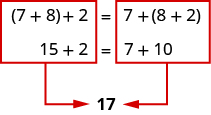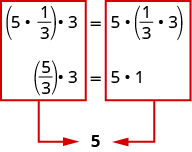## Rewriting Expressions Using the Commutative and Associative Properties

### Learning Outcomes

• Identify the associative and commutative properties of addition and multiplication
• Use the associative and commutative properties of addition and multiplication to rewrite algebraic expressions

## Use the Commutative and Associative Properties

Think about adding two numbers, such as $5$ and $3$.

$\begin{array}{cccc}\hfill 5+3\hfill & & & \hfill 3+5\hfill \\ \hfill 8\hfill & & & \hfill 8\hfill \end{array}$

The results are the same. $5+3=3+5$

Notice, the order in which we add does not matter. The same is true when multiplying $5$ and $3$.

$\begin{array}{cccc}\hfill 5\cdot 3\hfill & & & \hfill 3\cdot 5\hfill \\ \hfill 15\hfill & & & \hfill 15\hfill \end{array}$

Again, the results are the same! $5\cdot 3=3\cdot 5$. The order in which we multiply does not matter.

These examples illustrate the commutative properties of addition and multiplication.

### Commutative Properties

Commutative Property of Addition: if $a$ and $b$ are real numbers, then

$a+b=b+a$

Commutative Property of Multiplication: if $a$ and $b$ are real numbers, then

$a\cdot b=b\cdot a$

The commutative properties have to do with order. If you change the order of the numbers when adding or multiplying, the result is the same.

### example

Use the commutative properties to rewrite the following expressions:
1. $-1+3=$
2. $4\cdot 9=$

Solution:

 1. $-1+3=$ Use the commutative property of addition to change the order. $-1+3=3+\left(-1\right)$
 2. $4\cdot 9=$ Use the commutative property of multiplication to change the order. $4\cdot 9=9\cdot 4$

### try it

What about subtraction? Does order matter when we subtract numbers? Does $7 - 3$ give the same result as $3 - 7?$

$\begin{array}{ccc}\hfill 7 - 3\hfill & & \hfill 3 - 7\hfill \\ \hfill 4\hfill & & \hfill -4\hfill \\ & \hfill 4\ne -4\hfill & \end{array}$
The results are not the same. $7 - 3\ne 3 - 7$

Since changing the order of the subtraction did not give the same result, we can say that subtraction is not commutative.

Let’s see what happens when we divide two numbers. Is division commutative?

$\begin{array}{ccc}\hfill 12\div 4\hfill & & \hfill 4\div 12\hfill \\ \hfill \frac{12}{4}\hfill & & \hfill \frac{4}{12}\hfill \\ \hfill 3\hfill & & \hfill \frac{1}{3}\hfill \\ & \hfill 3\ne \frac{1}{3}\hfill & \end{array}$

The results are not the same. So $12\div 4\ne 4\div 12$

Since changing the order of the division did not give the same result, division is not commutative.

Addition and multiplication are commutative. Subtraction and division are not commutative.

Suppose you were asked to simplify this expression.

$7+8+2$

Some people would think $7+8\text{ is }15$ and then $15+2\text{ is }17$. Others might start with $8+2\text{ makes }10$ and then $7+10\text{ makes }17$.

Both ways give the same result, as shown below. (Remember that parentheses are grouping symbols that indicate which operations should be done first.)When adding three numbers, changing the grouping of the numbers does not change the result. This is known as the Associative Property of Addition.

The same principle holds true for multiplication as well. Suppose we want to find the value of the following expression:

$5\cdot \frac{1}{3}\cdot 3$

Changing the grouping of the numbers gives the same result.When multiplying three numbers, changing the grouping of the numbers does not change the result. This is known as the Associative Property of Multiplication.

If we multiply three numbers, changing the grouping does not affect the product.

You probably know this, but the terminology may be new to you. These examples illustrate the Associative Properties.

### Associative Properties

Associative Property of Addition: if $a,b$, and $c$ are real numbers, then

$\left(a+b\right)+c=a+\left(b+c\right)$

Associative Property of Multiplication: if $a,b$, and $c$ are real numbers, then

$\left(a\cdot b\right)\cdot c=a\cdot \left(b\cdot c\right)$

### example

Use the associative properties to rewrite the following:

1. $\left(3+0.6\right)+0.4=$
2. $\left(-4\cdot \frac{2}{5}\right)\cdot 15=$

### try it

Besides using the associative properties to make calculations easier, we will often use it to simplify expressions with variables.

### example

Use the Associative Property of Multiplication to simplify: $6\left(3x\right)$.

### try it

The following video provides more examples of how to simplify expressions using the commutative and associative properties of multiplication and addition.